# Introduction to Scikit-Learn in Machine Learning: A Complete Understanding

Machine learning and Artificial Intelligence have become some of the hottest topics among industries and academia. Every second person these days want to make a career in these domains. But the complete pipeline of machine learning solutions requires multiple levels of stack implementation. In such a scenario, machine learning enthusiasts require frameworks to make development and deployment easy in this area.

Scikit-learn is a free machine learning framework available for Python, providing an interface for supervised and unsupervised learning. Its free nature makes it more popular and accessible. It is built over the SciPy library and provides every feature catering to every ML system's requirement.

We will be using Scikit-learn for demonstrating and analyzing different models for our curriculum. So, this blog is to help you familiarize yourself with scikit-learn.

Note: This library has lots of tools and features, and we will be prioritizing certain features of this library over others as per our requirements. For installation-related instructions, you can refer to this beautiful blog.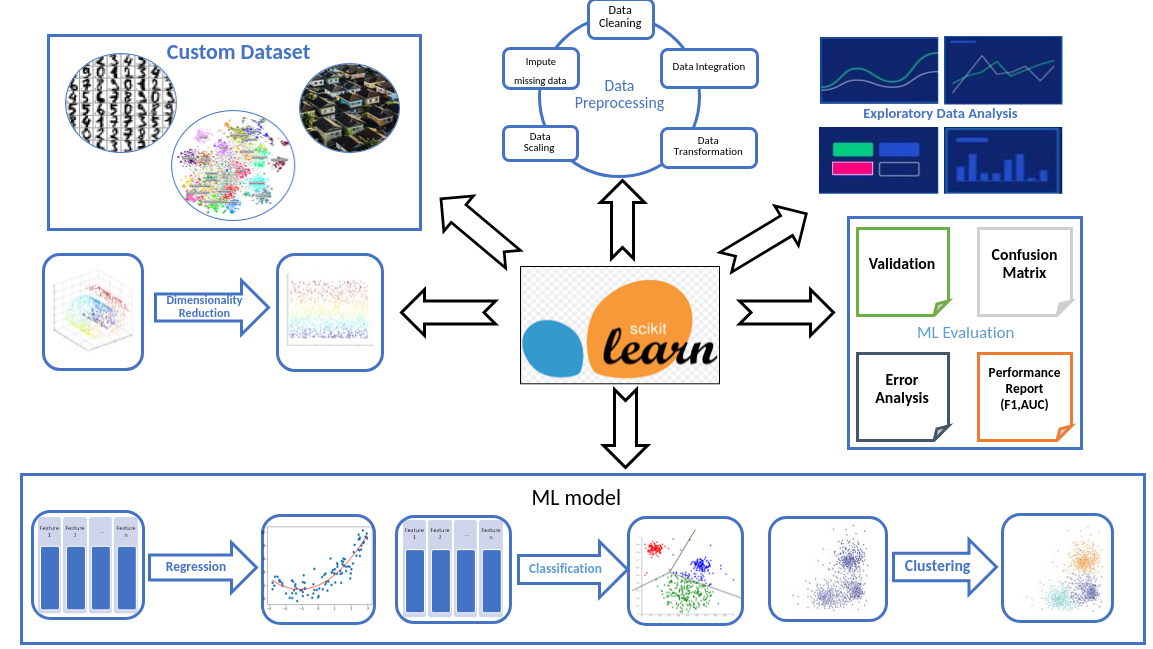### Data

Machine Learning starts with data, and while it is true that data and the purpose of using ML are user-specific. Still, learning ML has a lot to do with understanding model behavior on different datasets. To begin the journey in Machine Learning, the scikit-learn library provides a large set of data that are freely available as support and can be imported for the ML models.
There are different classes of the dataset, such as — toy dataset, that has some standard dataset, including

• Fisher-Iris dataset
• Hand-digit recognition,
• The breast cancer dataset, and
• the wine recognition dataset

Refer here to check out all the datasets in this category. Apart from the toy dataset, there are some real-world datasets incorporated in this library which comprises,

• Olivetti faces data-set from AT&T
• Newsgroups text

And similar datasets are prepared from real-world scenarios that we can find here. It even allows users to generate data randomly based on their requirement of testing the developed model.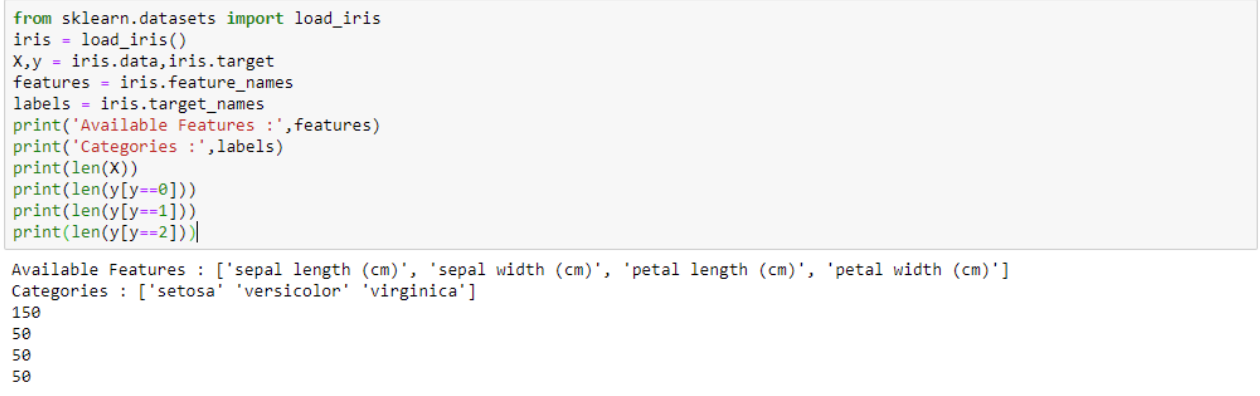The above code shows how to load and view the attributes of a sample dataset (iris flower) from sklearn.datasets. The total number of feature points in each category is shown in this code. Use the command dir(sklearn.datasets) to check all the datasets provided by this package. This package also offers the option to generate entirely new data.

• The functions make_moons or make_circles from sklearn.datasets can be used to generate 2-dimensional datasets that can be used for either clustering or classification models;
• make_classification can be used to generate datasets for classification models with any number of features and output class;
• make_regression can generate datasets for fitting regression models with any number of input features and informative features for generating output by a linear model.

Apart from these, several other datasets were provided by packages such as loadsvmlightfile, fetch_openml, etc.

### Pre-processing

The preprocessing stage of ML deals with obtaining data in a trainable format for the ML model. This requires,

• Selecting appropriate values for missing data
• Obtaining numerical values for categorical data,
• scaling attributes to improve training speed or accuracy.

#### Pre-processing: Imputing missing values

The sklearn library provides options to fill the missing values/outliers in a dataset. There can be several ways of replacing a missing value using the particular attribute's mean/median/mode. Several complex ML procedures use normalization/regularization to fill up these missing values. In this introductory section, however, we will only see the use of a simple imputer to replace missing values.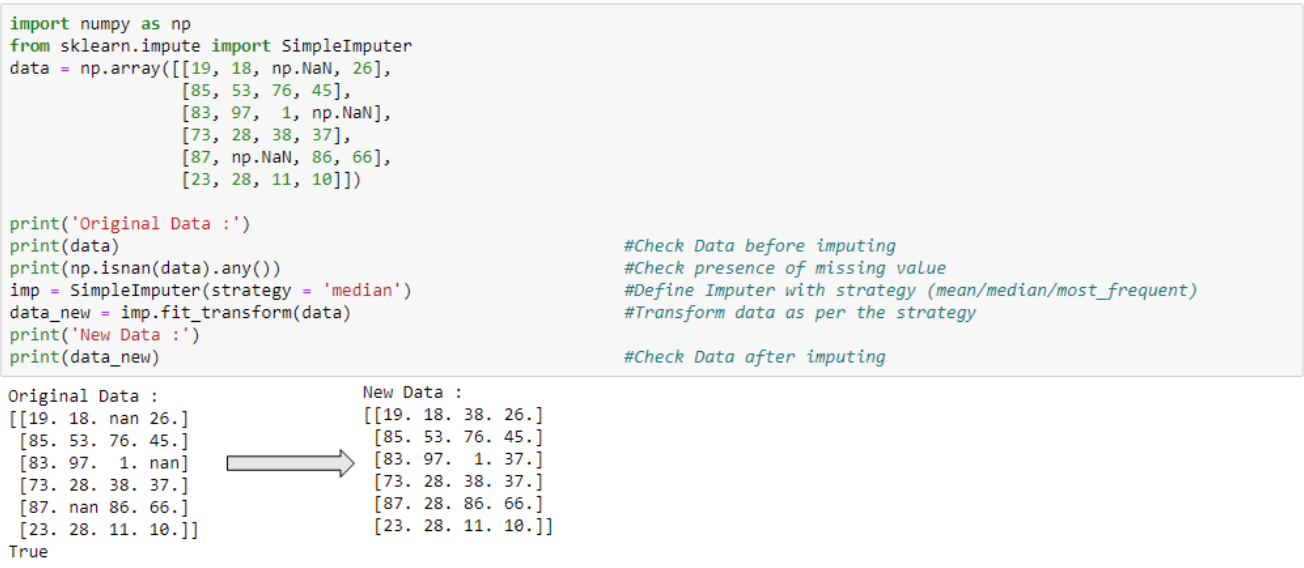Change the strategy to ‘mean’/‘most_frequent’ to replace the missing value with the attribute's mean or mode (column).

#### Pre-processing: Label Encoder

At times, the dataset available has specific values in the attribute which are non-numerical yet informative, and as such, these quantities cannot be processed by ML models. This is when a label encoder comes in handy. It can replace non-numerical portions with numerical amounts.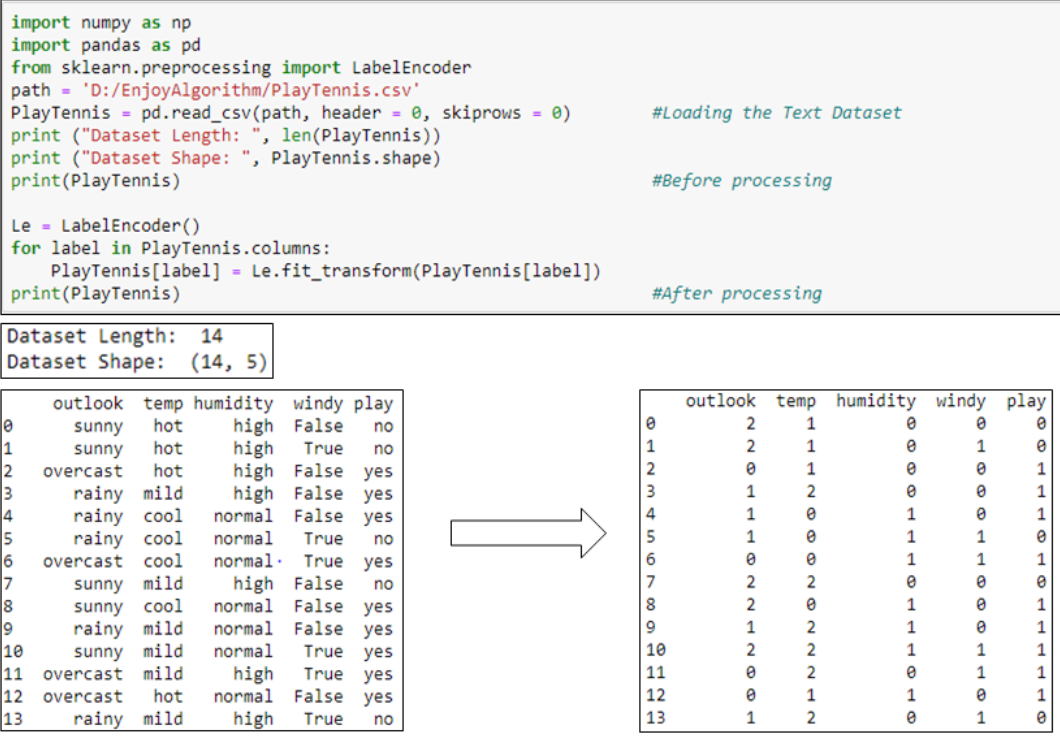For example, in the above playTennis dataset, the LabelEncoder assigned a numerical value to each non-numerical data entry (say ‘overcast’=0, ’rainy’=1, ‘sunny’=2). The processed data is now suitable for an ML model.

#### Pre-processing: Scaler

In many real-world datasets, the attributes are in different ranges. This can be a problem for an ML model as higher-order attributes can be preferred more (or less, depending on the algorithm). For example, a hiring manager has to develop a plan to propose the salary for an individual. Their only inputs are specific samples with previous wages and the number of years of work experience. Using an algorithm such as kNN, the attribute salary being in the higher range will outweigh the work experience (lower range). Thus to assign equal weightage of importance to both, a scaler is to be used.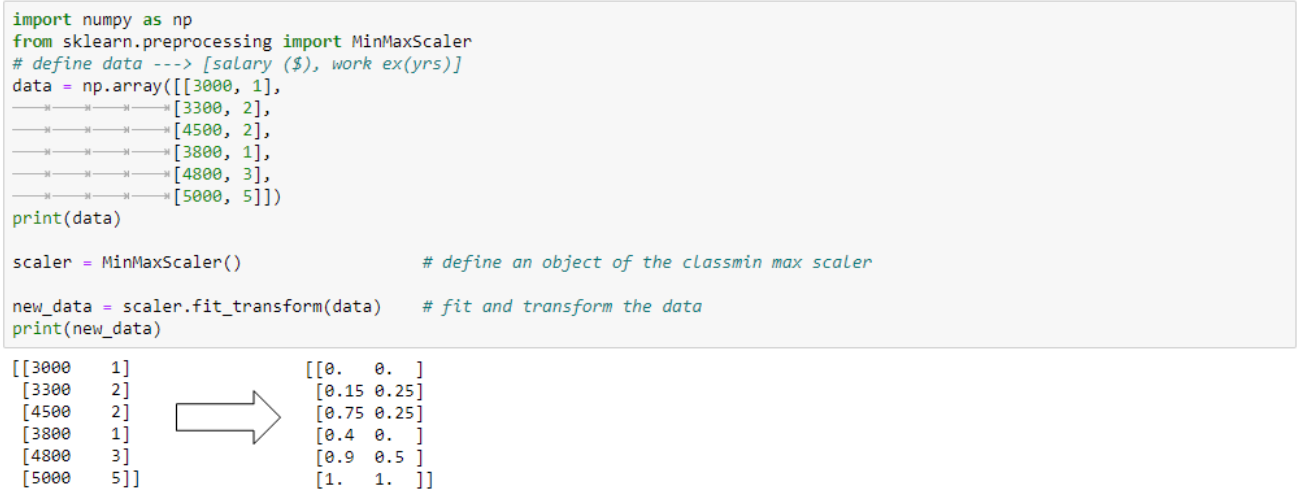There can be different scalars such as AbsScalar, StandardScalar which can serve purposes that are problem-specific.

### Feature Engineering

Feature engineering is the preparation of proper input for the available processable inputs. In short, taking whatever information we have for our problem and turning it into numbers can be used to build your feature matrix. This provides input well-suited with the machine learning algorithms.

#### Feature Engineering: Vectorization

Vectorization can expand any particular feature from the input having finite discrete possibilities. This step helps an ML model learn the individual importance of each category in the attribute.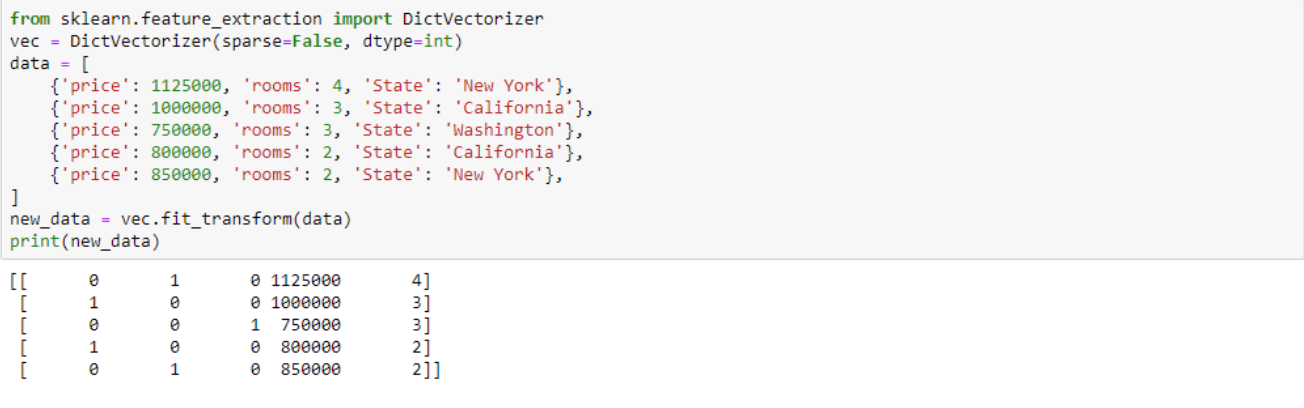Vectorization of the above data expanded the feature ‘state’ in the discrete categories provided in the dataset. This new data now add more meaning from the ML point of view.

#### Feature Engineering: Dimensionality Reduction

Dimensionality reduction is the projection of high-dimensional data to a low-dimensional space while retaining as much maximum variance. Datasets may have a significantly high number of attributes, and some may be redundant to the objective. Dimensionality reduction techniques can help remove such attributes as well as generate new attributes from them. PCA achieves dimensionality reduction by observing the co-relation among features. Let’s see how to use sklearn to reduce the dimensionality from 3 to 2 and the explained variance.As we can see, reducing the dimension from 3 → 2 still retains 0.759 (0.427+0.332)% of the variance of the 3-dimensional dataset. This is a very critical technique that has been used in several ML applications over the years.

### Building Machine Learning Model

The sklearn library provides several Machine Learning models classified based on their type (linear models, tree-based, SVM-based, ensemble-based, etc.). Some standard algorithms are shown below and how they are imported. Check out the complete list here.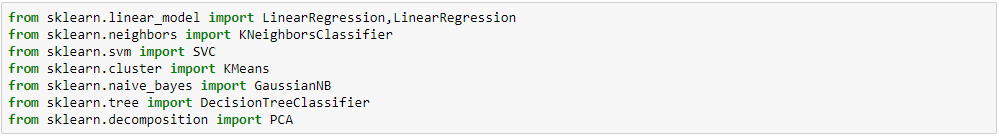#### The general paradigm for sklearn is

1. Import ML model and create an instance of it.
2. Fit training data into the model. For transformation or dimensionality reduction techniques, Fit and transform training data into the model.
3. Use the fitted model to predict.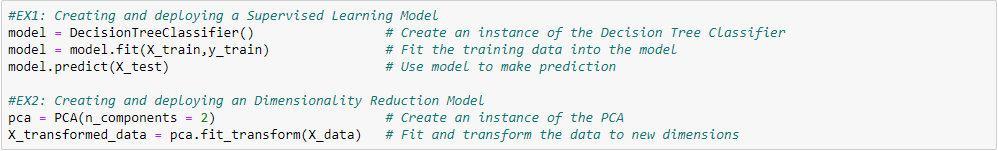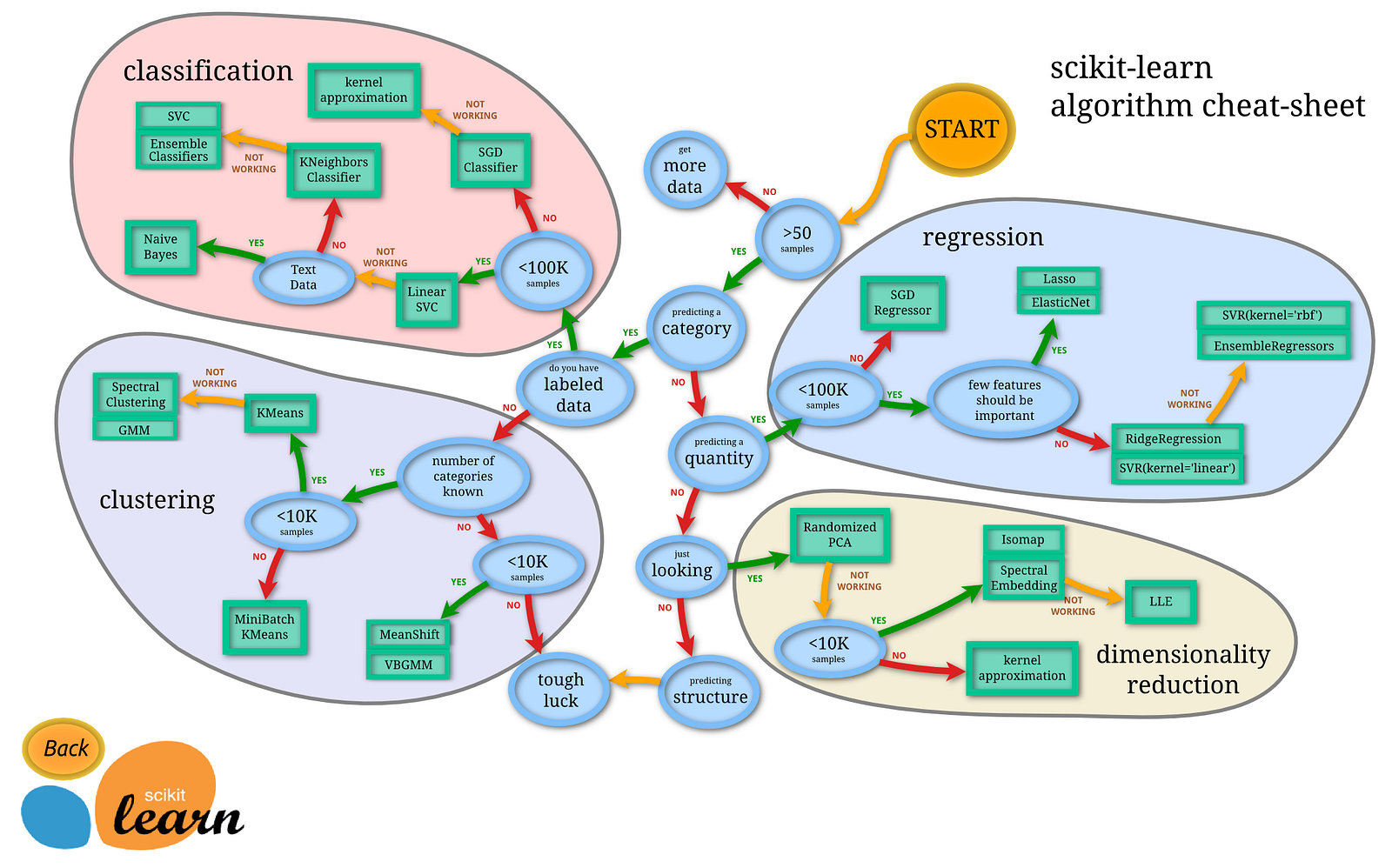Source: Scikit-learn.org

### Model Evaluation

At the beginner level, we are expected to understand different ML models and their performance on similar data. Occasionally, evaluate and compare models trained on similar data.

#### Evaluation of trained model can be done in two simple steps,

1. Import the desired metric
2. Compute performance using the test data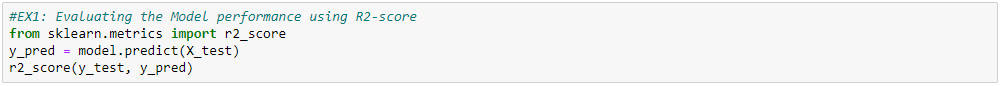### Building a complete Machine Learning Pipeline

So far, we have shown ways of extracting trainable data from raw data. This includes imputing missing values, transforming or scaling data, then using a model to train (fit) and predict outcomes. The same can be done in an organized, sequential way. A pipeline is a sequential application of transformation to generate a workflow, allowing processing and evaluating a model from end to end.

We will build an end-to-end pipeline using sklearn. We will be using the ‘iris’ flower dataset.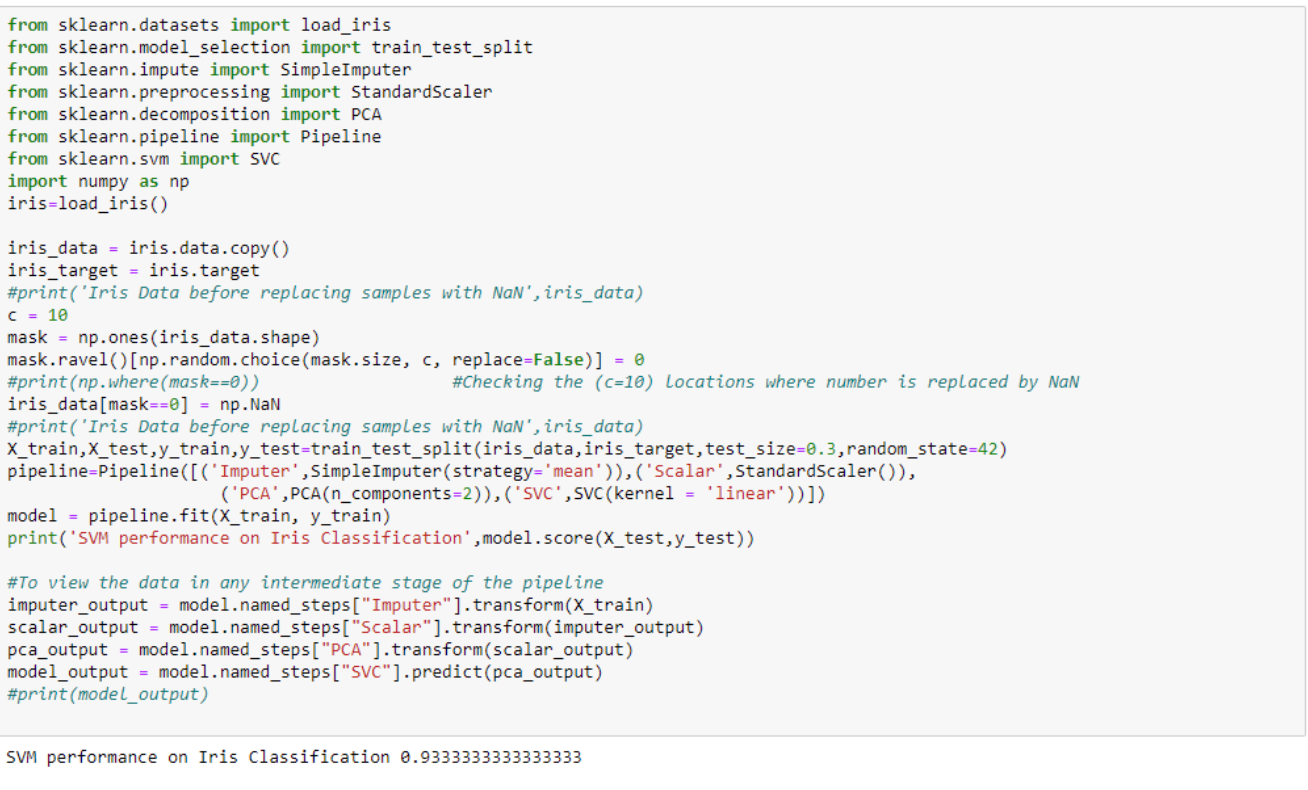To build the pipeline, we have to import Pipeline from sklearn.pipeline. This pipeline takes into input the different transformations that we chose to apply to our dataset. The iris dataset doesn’t have any missing values, so we will randomly replace a sample of values from the dataset with NaN (not a number). We replaced (c=10) values to NaN (use np.where() to check the locations of the matrix where this replacement is done).

Now that we have the data ready, we will split it into the training and testing dataset. Sklearn provides a feature ‘traintestsplit’ that can split the data into desired fractions.

The next step is building the pipeline. The pipeline takes in input as a list of tuples. The tuple indexed ‘0’ is the desired name for the transformation, and the indexed ‘1’ is the transformation to be applied. The pipeline consists of the following transformation,

#### Imputer →StandardScalar →PCA →SVM

1. The imputer handles the missing values as per the strategy. Feel free to change the strategy from ‘mean’ to ‘median’ or ‘most_frequent’ and check the results.
2. The StandardScalar transforms the data into zero mean and unit standard deviation. You can try other variations of scalars such as MinMaxScalar, MaxAbsScalar, etc.
3. PCA is used to reduce the dimensionality (4 →2) in this dataset.
4. Finally, the output from the PCA is fed into the SVC

We can then fit the pipeline into the training dataset and compute the accuracy on the test dataset. To view the output in any intermediate steps, use namedstep[“transformationname”] as shown in the code above. This will allow us to effectively see the pipeline results in the intermediate steps and understand how the pipeline is working.

### Conclusion

In this article, we have given an overview of how Scikit-learn plays an important role at every stage of Machine Learning. We discussed the datasets available, data pre-processing supports, feature engineering modules, fitting the desired model, and then learned about pipeline formation. Scikit-learn is a huge package, and what we have covered here is a tiny but valuable part to get started with. The content provided aligns with the stages of machine learning that we encounter and get deep into it.

### References

Scikit-learn: Machine Learning in Python, Pedregosa et al., JMLR 12, pp. 2825–2830, 2011.

Get well-designed application and interview centirc content on ds-algorithms, machine learning, system design and oops. Content will be delivered weekly.